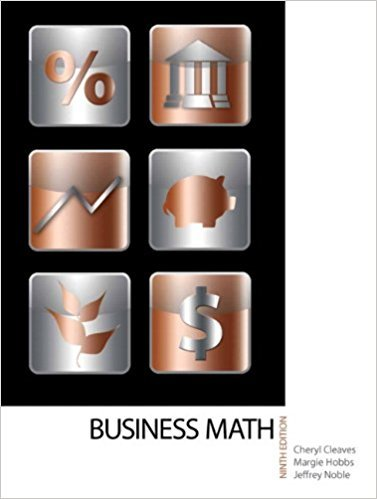×
×

# Use the first-in, first-out method to find the cost of goods sold and the cost of endingISBN: 9780135108178 355

## Solution for problem 6 Chapter 18

• Textbook Solutions
• 2901 Step-by-step solutions solved by professors and subject experts
• Get 24/7 help from StudySoup virtual teaching assistants4 5 1 384 Reviews
17
4
Problem 6

Use the first-in, first-out method to find the cost of goods sold and the cost of ending inventory using the table from Exercise 1 and the fact that the ending inventory is 96 units.

Step-by-Step Solution:
Step 1 of 3

L35 - 11 3 ex. The concentration C (in mg/cm )o fadugi a- tient’s bloodstream t hours after an injection is decreasing...

Step 2 of 3

Step 3 of 3

##### ISBN: 9780135108178

The answer to “Use the first-in, first-out method to find the cost of goods sold and the cost of ending inventory using the table from Exercise 1 and the fact that the ending inventory is 96 units.” is broken down into a number of easy to follow steps, and 34 words. This textbook survival guide was created for the textbook: Business Math, , edition: 9. Since the solution to 6 from 18 chapter was answered, more than 239 students have viewed the full step-by-step answer. The full step-by-step solution to problem: 6 from chapter: 18 was answered by , our top Math solution expert on 03/08/18, 08:36PM. Business Math, was written by and is associated to the ISBN: 9780135108178. This full solution covers the following key subjects: . This expansive textbook survival guide covers 77 chapters, and 1559 solutions.

Unlock Textbook Solution